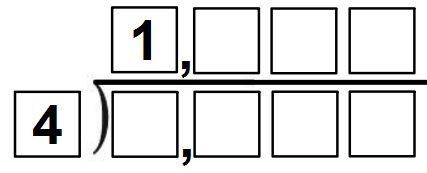# Division Fill in the Blanks (no remainder)

Directions: Fill in the blanks using all different non-zero digits (except the numbers 1 and 4, which have already been used) to make the greatest possible quotient.### Hint

What number should ideally be placed in the hundreds place of the quotient? Why?
Can the units’ digit of the dividend be odd if the quotient has no remainder?

1,963 (the dividend is 7,852)

Source: Brian Lack

## Product Close to 1,000

Directions: Using the digits 1 to 9 at most one time each, place a digit …

1.Should have added as an additional hint, “can the units digit of the dividend be odd if the quotient has no remainder?”

•Robert Kaplinsky

2.3.1963 (the dividend is 7852

4.1963 the dividend is 7852

5.1,000 \ 2 = 500

6.the answer to this problem is 1963 and the divedended is 7852.Because 4×1=4 9×4=36 6×4=24 3×4=12

•i thinks she is correct

7.hard! figured out tho 😀

8.I have a student that found another solution – 6,952 divided by 4 = 1,738